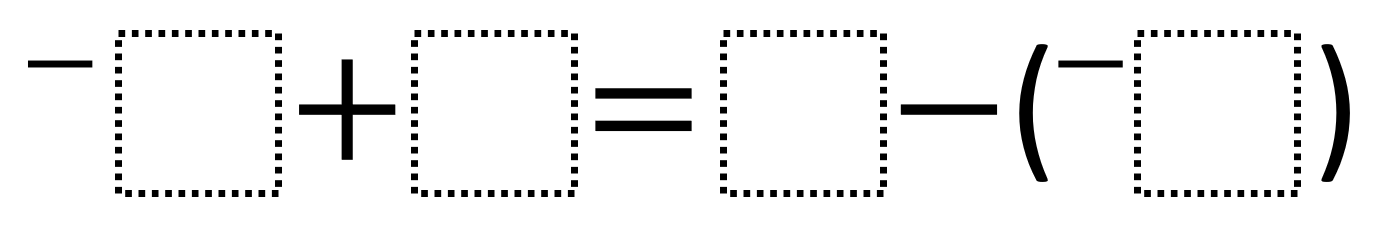# Adding And Subtracting Rational Numbers 2

Directions: Using the integers -9 to 9 at most one time each, fill in the boxes to create an equation where each side has the greatest possible value.### Hint

How can you verify the value of each side?

### Answer

The greatest possible value is 16 and can be gotten through an equation like –-8 + 8 = 9 – (-7)

Source: Robert Kaplinsky

## Complimentary and Supplementary Angles 2

Directions: Using the digits 0 to 9 at most one time each, fill in the …